StatLect

Empirical distribution

The empirical distribution, or empirical distribution function, can be used to describe a sample of observations of a given variable. Its value at a given point is equal to the proportion of observations from the sample that are less than or equal to that point.Definition

The following is a formal definition.

Definition Letbe a sample of size, where,...,are theobservations from the sample. The empirical distribution function of the sampleis the functiondefined aswhereis an indicator function that is equal toifandotherwise.

In other words, the value of the empirical distribution function at a given pointis obtained by:

1. counting the number of observations that are less than or equal to;

2. dividing the number thus obtained by the total number of observations, so as to obtain the proportion of observations that is less than or equal to.

An example follows.

Example Suppose we observe a sample made of four observations:whereWhat is the value of the empirical distribution function of the sampleat the point? According to the definition above, it is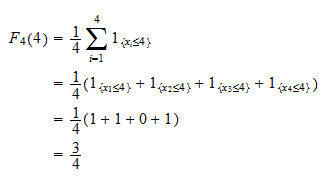In other words, the proportion of observations that are less than or equal tois.

The empirical distribution is the distribution function of a discrete variable

Let,...,be the sample observations ordered from the smallest to the largest (in technical terms, the order statistics of the sample).

Then it is easy to see that the empirical distribution function can be written as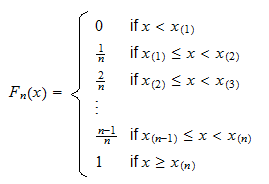This is a function that is everywhere flat except at sample points, where it jumps by. It is the distribution function of a discrete random variablethat can take any one of the values,...,with probability. In other words, it is the distribution function of a discrete variablehaving probability mass function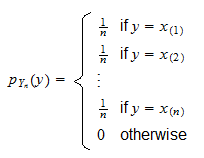Finite sample properties

When theobservations from the sample,...,are the realizations ofrandom variables,...,, then the valuetaken by the empirical distribution at a given pointcan also be regarded as a random variable. Under the hypothesis that all the random variables,...,have the same distribution, the expected value and the variance ofcan be easily computed, as shown in the following proposition.

Proposition If theobservations in the sampleare the realizations ofrandom variables,...,having the same distribution function, thenfor any. Furthermore, if,...,are mutually independent, thenfor any.

Proof

The result about the expected value is proved by using the definition of distribution function and the properties of indicator functions (in particular, the fact that the expected value of an indicator is equal to the probability of the event it indicates):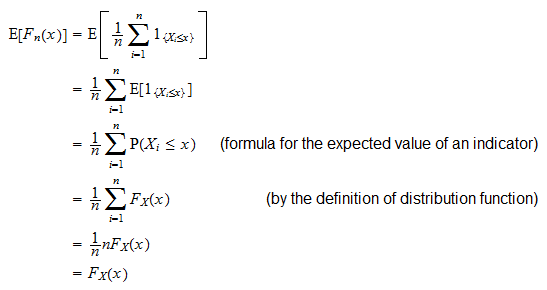The result about the variance is proved as follows: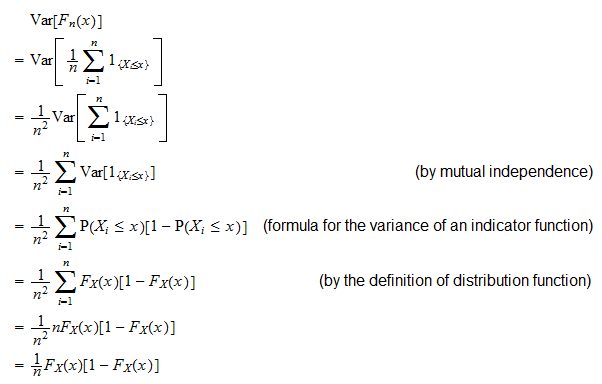Thus, for any given point, the empirical distribution function is an unbiased estimator of the true distribution function. Furthermore, its variance tends to zero as the sample size becomes large (astends to infinity).

Large sample properties

An immediate consequence of the previous result is that the empirical distribution converges in mean-square to the true one.

Proposition If theobservations in the sampleare the realizations ofmutually independent random variables,...,having the same distribution function, thenfor any.

Proof

We have that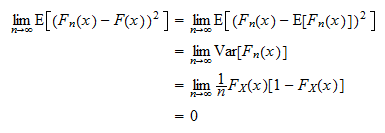As a matter of fact, it is possible to prove a much stronger result, called Glivenko-Cantelli theorem, which states that not onlyconverges almost surely tofor each, but it also converges uniformly, that is,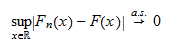Furthermore, the assumption that the random variables,...,be mutually independent can be relaxed (see, e.g., Borokov 1999) to allow for some dependence among the observations (similarly to what can be done for the Law of Large Numbers; see Chebyshev's Weak Law of Large Numbers for correlated sequences).

References

Borokov, A. A. (1999) Mathematical statistics, CRC Press.

The book

Most of the learning materials found on this website are now available in a traditional textbook format.

Glossary entries
Share